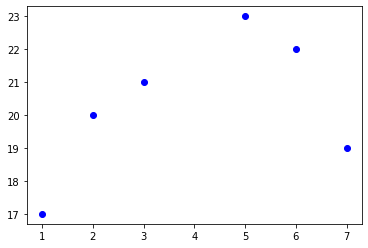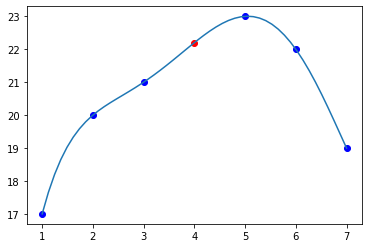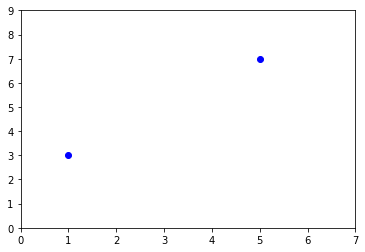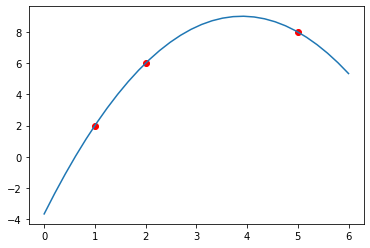# 插值法

## 什么是插值## 为什么要插值

$P_n(x) = a_0 + a_1x + a_2x^2 + a_3x^3 + a_4x^4 + a_5x^5$

$a_0,a_1,a_2,a_3,a_4,a_5$

$\begin{cases}a_0 + a_1x_1 + a_2x_1^2 + a_3x_1^3 + a_4x_1^4 + a_5x_1^5 = y_1\\a_0 + a_1x_2 + a_2x_2^2 + a_3x_2^3 + a_4x_2^4 + a_5x_2^5 = y_2\\a_0 + a_1x_3 + a_2x_3^3 + a_3x_3^3 + a_4x_3^4 + a_5x_3^5 = y_3\\a_0 + a_1x_4 + a_2x_4^2 + a_3x_4^3 + a_4x_4^4 + a_5x_4^5 = y_4\\a_0 + a_1x_5 + a_2x_5^2 + a_3x_5^3 + a_4x_5^4 + a_5x_5^5 = y_5\\a_0 + a_1x_6 + a_2x_6^2 + a_3x_6^3 + a_4x_6^4 + a_5x_6^5 = y_6\\\end{cases}$

$\begin{bmatrix}1 & x_1 & x_1^2 & x_3^3 & x_4^4 & x_5^5\\1 & x_2 & x_2^2 & x_2^3 & x_2^4 & x_2^5\\1 & x_3 & x_3^2 & x_3^3 & x_3^4 & x_3^5\\1 & x_4 & x_4^2 & x_4^3 & x_4^4 & x_4^5\\1 & x_5 & x_5^2 & x_5^3 & x_5^4 & x_5^5\\1 & x_6 & x_6^2 & x_6^3 & x_6^4 & x_6^5\\\end{bmatrix}\begin{bmatrix}a_0 \\ a_1 \\ a_2 \\ a_3 \\ a_4 \\ a_5 \\\end{bmatrix}=\begin{bmatrix}y_1 \\ y_2 \\ y_3 \\ y_4 \\ y_5 \\ y_6 \\\end{bmatrix}$

$det(V)\neq0$

## 拉格朗日插值法

#### 核心思想

$P(x_1)=y_1, P(x_2)=y_2, P(x_i)=y_i$，于是构造出这样的一种形式

$P(x) = y_1 L_1(x) + y_2 L_2(x) + \dots y_i L_i(x)$

$x=x_1$时， 要让$P(x_1) = y_1L_1(x_1) + y_2L_2(x_1)+\dots y_iL_i(x_i) = y1$

$\begin{cases}L_i(x_i) = 1\\L_i(x_j) = 0 (j \neq i)\end{cases}$

#### 1.线性插值$y = \frac{(y_1-y_0)}{x_1-x_0}(x-x_0) + y_0$

\begin{align}y &= \frac{y_1 - y_0}{x_1 - x_0}(x-x_0) + y_0\\ &= y_1\frac{x-x_0}{x_1-x_0} + y_0\frac{x-x_0}{x_0-x_1} + y_0\\ &= y_0\frac{x-x_1}{x_0-x_1} + y_1\frac{x-x_0}{x_1-x_0}\end{align}

$P(x) = y_0\frac{x-x_1}{x_0-x_1} + y_1\frac{x-x_0}{x_1-x_0}$

$L_0(x) = \frac{x-x_1}{x_0-x_1}\\L_1(x) = \frac{x-x_0}{x_1-x_0}$

#### 2.抛物插值$P(x) = y_0L_0(x) + y_1L_1(x) + y_2L_2(x)$

• 当分子为0，不论分母取什么，整个分式都为0

• 当分子不为0，如果分子和分母相等，则分式等于1

$L_0(x) = \frac{(x-x_1)(x-x_2)}{(x_0-x_1)(x_0-x_2)}\\L_1(x) = \frac{(x-x_0)(x-x_2)}{(x_1-x_0)(x_1-x_2)}\\L_2(x) = \frac{(x-x_0)(x-x_1)}{(x_2-x_0)(x_2-x_1)}$

$P(x) = y_0 \frac{(x-x_1)(x-x_2)}{(x_0-x_1(x_0-x_2)}+y_1 \frac{(x-x_0)(x-x_2)}{(x_1-x_0)(x_1-x_2)} +y_2 \frac{(x-x_0)(x-x_1)}{(x_2-x_0)(x_2-x_1)}$

#### 3.多项式插值（一般情形）

$P(x) = y_0L_0(x) + y_1L_1(x) + y_2L_2(x) + y_3L_3(x) + y_4L_4(x) + y_5L_5(x)$

$L_0(x) = \frac{(x-x_1)(x-x_2)(x-x_3)(x-x_4)(x-x_5)}{(x_0-x_1)(x_0-x_2)(x_0-x_3)(x_0-x_4)(x_0-x_5)}\\L_1(x) = \frac{(x-x_0)(x-x_2)(x-x_3)(x-x_4)(x-x_5)}{(x_1-x_0)(x_1-x_2)(x_1-x_3)(x_1-x_4)(x_1-x_5)}\\L_2(x) = \frac{(x-x_0)(x-x_1)(x-x_3)(x-x_4)(x-x_5)}{(x_2-x_0)(x_2-x_1)(x_2-x_3)(x_2-x_4)(x_2-x_5)}\\L_3(x) = \frac{(x-x_0)(x-x_1)(x-x_2)(x-x_4)(x-x_5)}{(x_3-x_0)(x_3-x_1)(x_3-x_2)(x_3-x_4)(x_3-x_5)}\\L_4(x) = \frac{(x-x_0)(x-x_1)(x-x_2)(x-x_3)(x-x_5)}{(x_4-x_0)(x_4-x_1)(x_4-x_2)(x_4-x_3)(x_4-x_5)}\\L_5(x) = \frac{(x-x_0)(x-x_1)(x-x_2)(x-x_3)(x-x_4)}{(x_5-x_0)(x_5-x_1)(x_5-x_2)(x_5-x_3)(x_5-x_4)}\\$

$L_i(x) = \prod_{j=0,j\neq k}^{n} \frac{x-x_j}{x_i - x_j}\\P_n(x) = \sum_{i=0}^{n}y_iL_i(x)$

#### 4.编程实现

import numpy as np
from matplotlib import pyplot as plt

''' 关键代码 '''
def Lagrange(X,Y,x):   #X是观测点的横坐标数组，Y是纵坐标数组,x是要求的点的横坐标（可以是数组）
n = len(X)          #n个观测点，对应n-1次插值
y = 0               #y的初值设为0
for i in range(n):
L = 1           #把插值基函数初始化为1
for j in range(n):
if(i!=j):
L*=(x-X[j])/(X[i]-X[j]) #按照刚刚的公式算出个分式然后累乘
y+=Y[i]*t    #然后是累加
return y

'''调用一下看看'''
X = np.array([1,2,3,5,6,7])
Y = np.array([17,20,21,23,22,19])
x = np.linspace(1,7,50)  #生成区间1到7等距的50个值
y = Lagrange(X,Y,x)     #求出对于的50个插值
plt.plot(X,Y,'ob',x4,y4,'or',x,y)  #画图



### 牛顿插值法（太懒了，先歇会儿）

posted @ 2020-03-29 18:20  裏表異体  阅读(52)  评论(0编辑  收藏